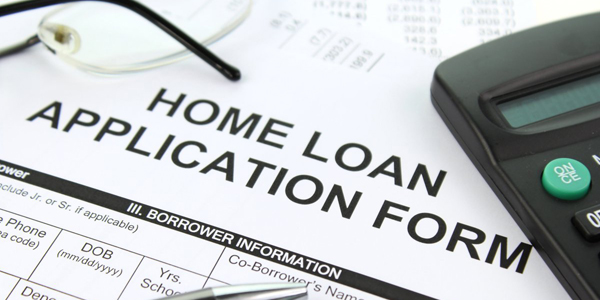Home / Money Blog

## Affording that house - monthlyNote: You can use any financial calculator to do this problem, but if you want the BEST, you can get our 10bii Financial Calculator for iOS, Android, Mac, and Windows!THE SCENARIO Last time, I talked a bit about rising interest rates and their affect on the size of a loan a person can afford. Today, I'd like to take a look at the same question, but from a different angle. So this time, we're going to assume that I want to buy a \$500,000 house, and I have 20% (\$100,000) to put down when I complete the purchase. I'm going to assume that I can get a 30-year, fully-amortizing loan for the remaining \$400,000 balance. The question: How much higher would my monthly payment be if I were to borrow the money at 5.25% instead of 4.125%?
THE SOLUTION This question is relatively straightforward: figure out the monthly payment at two different interest rates, and compare the two numbers. First things first, make sure the calculator is using 12 Payments per Year. Loan 1: Borrowing at 4.125% N: 360 (I'm getting a 30-year loan) I/YR: 4.125 (I'm borrowing the money at 4.125%) PV: 400,000 (I'm borrowing \$400,000) PMT: (This is what I'm trying to find) FV: 0 (The loan amortizes fully)

If I borrow the money at 4.125%, my monthly payment will be \$1,938.60.

Loan 2: Borrowing at 5.25% N: 360 (I'm getting a 30-year loan) I/YR: 5.25 (I'm borrowing the money at 5.25%) PV: 400,000 (I'm borrowing \$400,000) PMT: (This is what I'm trying to find) FV: 0 (The loan amortizes fully)

If I borrow the money at 5.25%, my monthly payment will be \$2,208.81.